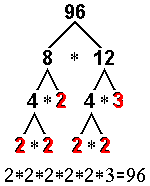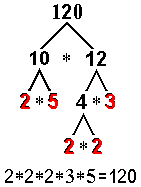# Properties of NumbersPrime FactorizationThere are different methods which can be utilized to find the prime factorization of a number. One way is to repeatedly divide by prime numbers: EXAMPLE   1.   Prime factorization of 96 (by division): 96 ÷ 2 = 48     48 ÷ 2 = 24         24 ÷ 2 = 12             12 ÷ 2 = 6                 6 ÷ 2 = 3                     3 ÷ 3 = 1 96 = 2 * 2 * 2 * 2 * 2 * 3 EXAMPLE   2.   Prime factorization of 120 (by division): 120 ÷ 2 = 60     60 ÷ 2 = 30         30 ÷ 2 = 15             15 ÷ 3 = 5                 5 ÷ 5 = 1 120 = 2 * 2 * 2 * 3 * 5 Another way to approach the task is to choose ANY pair of factors and split these factors until all the factors are prime:Traditionally, the factors are listed in order from least to greatest. While the product would be the same regardless of the order of the factors, it is much easier to compare or evaluate expressions if the factors are listed in this order. Determining the prime factors can be challenging. While it's good to know HOW to do it, on most standardized tests you'll only have to select the one right answer choice to show that you understand the concept. The CORRECT answer:         must be only PRIME numbers         must multiply together to give the specified quantity.